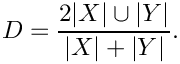plastimatch
Dice_statistics Class Reference

The Dice_statistics class computes a Dice statistic for the overlap between two regions. Dice is defined asA value of zero means X and Y have no overlap, where a value of one means the two regions are the same. More...

#include <dice_statistics.h>

## Public Member Functions

Dice_statistics ()

~Dice_statistics ()

Inputs
void set_reference_image (const char *image_fn)
Set the reference image. The image will be loaded from the specified filename. More...

void set_reference_image (const UCharImageType::Pointer &image)
Set the reference image as an ITK image. More...

void set_compare_image (const char *image_fn)
Set the compare image. The image will be loaded from the specified filename. More...

void set_compare_image (const UCharImageType::Pointer &image)
Set the compare image as an ITK image. More...

Execution
void run ()
Compute dice statistics. More...

Outputs
float get_dice ()
Return the Dice coefficient value. More...

float get_sensitivity ()
Return sensitivity coefficient value. More...

float get_specificity ()
Return specificity coefficient value. More...

size_t get_true_positives ()
Return the number of true positive voxels, i.e. positive reference voxels that are positive in compare image. More...

size_t get_true_negatives ()
Return the number of true negative voxels, i.e. negative reference voxels that are negative in compare image. More...

size_t get_false_positives ()
Return the number of false positive voxels, i.e. negative reference voxels that are positive in compare image. More...

size_t get_false_negatives ()
Return the number of false negative voxels, i.e. positive reference voxels that are negative in compare image. More...

DoubleVector3DType get_reference_center ()
Return the location of the center of mass of the reference image structure. More...

DoubleVector3DType get_compare_center ()
Return the location of the center of mass of the compare image structure. More...

double get_reference_volume ()
Return the volume of the reference image structure. More...

double get_compare_volume ()
Return the volume of the compare image structure. More...

void debug ()
Display debugging information to stdout. More...

## Public Attributes

Dice_statistics_private * d_ptr

## Detailed Description

The Dice_statistics class computes a Dice statistic for the overlap between two regions. Dice is defined asA value of zero means X and Y have no overlap, where a value of one means the two regions are the same.

If the images do not have the same size and resolution, the compare image will be resampled onto the reference image geometry prior to comparison.

## ◆ Dice_statistics()

 Dice_statistics::Dice_statistics ( )

## ◆ ~Dice_statistics()

 Dice_statistics::~Dice_statistics ( )

## ◆ debug()

 void Dice_statistics::debug ( void )

Display debugging information to stdout.

## ◆ get_compare_center()

 DoubleVector3DType Dice_statistics::get_compare_center ( )

Return the location of the center of mass of the compare image structure.

## ◆ get_compare_volume()

 double Dice_statistics::get_compare_volume ( )

Return the volume of the compare image structure.

## ◆ get_dice()

 float Dice_statistics::get_dice ( )

Return the Dice coefficient value.

## ◆ get_false_negatives()

 size_t Dice_statistics::get_false_negatives ( )

Return the number of false negative voxels, i.e. positive reference voxels that are negative in compare image.

## ◆ get_false_positives()

 size_t Dice_statistics::get_false_positives ( )

Return the number of false positive voxels, i.e. negative reference voxels that are positive in compare image.

## ◆ get_reference_center()

 DoubleVector3DType Dice_statistics::get_reference_center ( )

Return the location of the center of mass of the reference image structure.

## ◆ get_reference_volume()

 double Dice_statistics::get_reference_volume ( )

Return the volume of the reference image structure.

## ◆ get_sensitivity()

 float Dice_statistics::get_sensitivity ( )

Return sensitivity coefficient value.

## ◆ get_specificity()

 float Dice_statistics::get_specificity ( )

Return specificity coefficient value.

## ◆ get_true_negatives()

 size_t Dice_statistics::get_true_negatives ( )

Return the number of true negative voxels, i.e. negative reference voxels that are negative in compare image.

## ◆ get_true_positives()

 size_t Dice_statistics::get_true_positives ( )

Return the number of true positive voxels, i.e. positive reference voxels that are positive in compare image.

## ◆ run()

 void Dice_statistics::run ( )

Compute dice statistics.

## ◆ set_compare_image() [1/2]

 void Dice_statistics::set_compare_image ( const char * image_fn )

Set the compare image. The image will be loaded from the specified filename.

## ◆ set_compare_image() [2/2]

 void Dice_statistics::set_compare_image ( const UCharImageType::Pointer & image )

Set the compare image as an ITK image.

## ◆ set_reference_image() [1/2]

 void Dice_statistics::set_reference_image ( const char * image_fn )

Set the reference image. The image will be loaded from the specified filename.

## ◆ set_reference_image() [2/2]

 void Dice_statistics::set_reference_image ( const UCharImageType::Pointer & image )

Set the reference image as an ITK image.

## ◆ d_ptr

 Dice_statistics_private* Dice_statistics::d_ptr

The documentation for this class was generated from the following files: